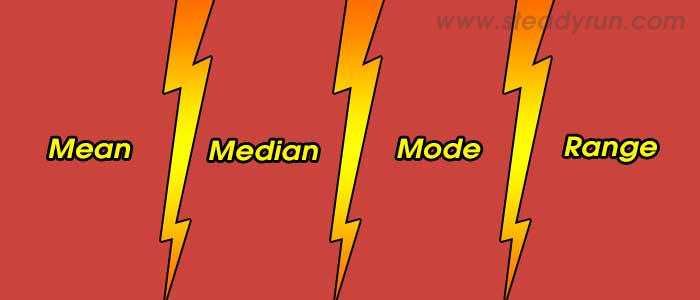# Difference between Mean, Median, Mode and RangeDistinguish, differentiate, compare and explain what is the difference between Mean, Median, Mode and Range. Comparison and Differences.

## Difference between Mean, Median, Mode and Range

To find the mean, median, mode and range firts we have to arrange the given sequence of numbers in increasing order.

For example: Let the sequence be 2, 5, 3, 8, 7, 9, 3, 7, 12, 4

Then after sorting the numbers in increasing order, the sequences becomes: 2, 3, 3, 4, 5, 7, 7, 8, 9, 12

## Mean

Mean can be calculated as the average of the numbers.

To find the average:

1. First add all numbers in the series.

2. Divide the sum by how many numbers were added.

Example: 2 + 3 + 3 + 4 + 5 + 7 + 7 + 8 + 9 + 12 = 60

Mean = 60 / 10 = 6

## Median

Median is the middle numbe rof the sequence.

1. The median is the middle number when numbers are arrange in order by size.

2. If the sequence contains even number of numbers than the median is the average of tw numbers in the middle.

Median = (5 + 7) / 2 = 6

## Mode

Mode is the number that occures most often in the sequence.

1. Find the numbers that occures most often in the sequence. (there may be more than one)

Mode = 3 and 7

## Range

Range is the difference between the highest and smallest value in the sequence.

Range = 12 - 2 = 10

#### Tags:

Difference between Range vs Mean, Median, Mode

Mean, Median, Mode vs Range

Differences between Range vs Mean, Median, Mode

### Latest Articles### Spreading Knowledge Across the World

USA - United States of America  Canada  United Kingdom  Australia  New Zealand  South America  Brazil  Portugal  Netherland  South Africa  Ethiopia  Zambia  Singapore  Malaysia  India  China  UAE - Saudi Arabia  Qatar  Oman  Kuwait  Bahrain  Dubai  Israil  England  Scotland  Norway  Ireland  Denmark  France  Spain  Poland  and  many more....